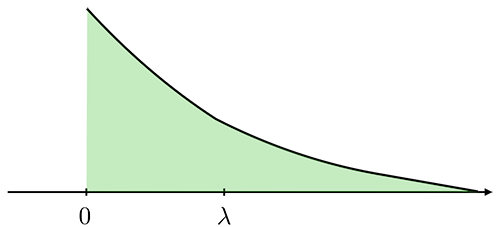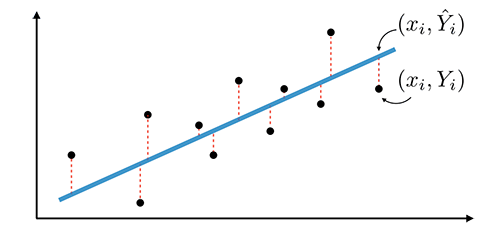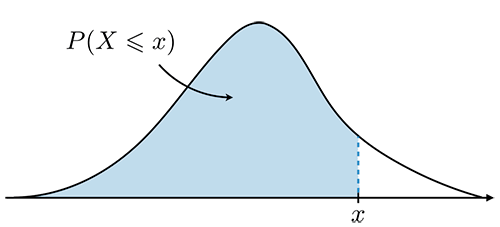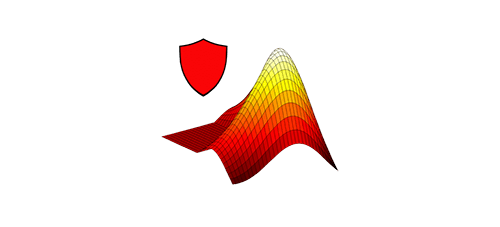Teaching

### CME 106 ― Introduction to Probability and Statistics for Engineers Star

My twin brother Afshine and I created this set of cheatsheets when I was a TA for Stanford's CME 106 class in Winter 2018. They can (hopefully!) be useful to all future students taking this course as well as to anyone else interested in learning the fundamentals of Probabilities and Statistics.

#### Cheatsheet

Probability• • Basics and probability distributions
• • Random variables, expectation and moments
• • Conditional probability, joint distributions
Statistics• • Mean and variance estimation
• • Confidence intervals, hypothesis testing
• • Regression and correlation analysis

#### Takeaways

Key concepts• • Using a distribution table
• • Mean and variance confidence intervals
Distribution tables• • Gaussian distribution
• • Student's t-distribution
• • Chi-squared distribution
Common questions• • Definition of poker hands

#### Matlab tips

Getting started• • Installation and first steps
• • Built-in functions, vectors, matrices, operators
• • Functions, plots and good practices
Course functionalities• • Discrete distribution functions: uniform, binomial, Poisson
• • Continuous distribution functions: uniform, normal, exponential